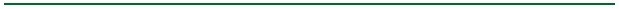Fundamentals of Queueing Theory Fifth Edition John F. Shortle James M. Thompson Donald Gross Carl M. Harris (c) 2018 by John Wiley & Sons, Inc, Hoboken, NJ.To install: Create a directory to contain the software (name of your choosing),

For information on previous edition, see Fundamentals of Queueing Theory, 4th edition• Chapter 1: Introduction
• Chapter 2: Review of Stochastic Processes
• Chapter 3: Simple Markovian Queueing Models
• Chapter 4: Advanced Markovian Queueing Models
• Chapter 5: Networks, Series, and Cyclic Queues
• Chapter 6: General Arrival or Service Patterns
• Chapter 7: General Models and Theoretical Topics
• Chapter 8: Bounds and Approximations
• Chapter 9: Numerical Techniques and Simulation

Key Changes From 4th Edition

• Chapter 1: Introduction
• Chapter 1 has been split into two chapters (1 and 2), with substantial editing, reorganization, and new material
• New and expanded section on Little's law
• New section on the experience of waiting
• Chapter 2: Review of Stochastic Processes
• Expanded material (previously in Chapter 1), substantially reorganized and revised
• Many new figures and examples
• Chapter 3 Advanced Markovian Queueing Models
• New sub-section on fairness in queueing
• New material on processor sharing
• Chapter 8 Numerical Techniques and Simulation
• New section on deterministic fluid queues
• Over 60 new problems and 20 new examples throughout text
• Editing for clarity throughout text
• QtsPlus software has been updated with significantly improved user interface

Errata

• p. 11. In equation (1.1), the superscript for W(·) in the middle equation is wrong: W(k) should be W(i). See also the next bullet.
• p. 17. In the equation preceding Theorem 1.2, the superscript for G(·) in the middle equation is wrong: G(k) should be G(i).
• p. 96. The left-hand side of the first equation on the page should be 'Pr{Tt | Tq > 0} = ', not 'Pr{Tt} = '. This is used to obtain the next equation for W(t), which can be written as W(t) = Pr{Tq = 0} Pr{Tt | Tq = 0} + Pr{Tq > 0} Pr{Tt | Tq > 0}. The equation for W(t) is correct.